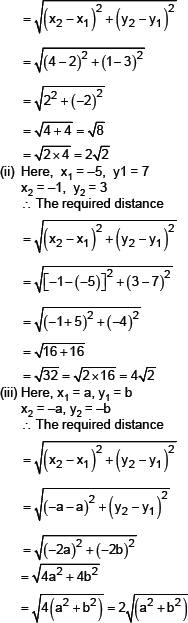#### EXERCISE

##### Type - 4
###### Question 1 :
On dividing x– 3x2 + x + 2 by a polynomial g(x), the quotient and remainder were x–2 and -2x + 4 respectively. Find g(x).
###### Formulae used :

Distance Formula to find distance between two points (x1,y1) and (x2,y2) is :                                                D = √[(x2 – x1)2 + (y2 – y1)2 ]

###### Solution :
Sol. (i) Here x1 = 2, y1 = 3, x2 = 4 and y2 = 1
∴ The required distance###### Class Work
Q.1.   Find the distance between the  following pairs of points:
(i)   (2, 3), (4, 1)
(ii)   (–5, 7), (–1, 3)
(iii)  (a, b), (–a, –b)
Q.1.   Find the distance between the  following pairs of points:
(i)   (2, 3), (4, 1)
(ii)   (–5, 7), (–1, 3)
(iii)  (a, b), (–a, –b)
Q.1.   Find the distance between the  following pairs of points:
(i)   (2, 3), (4, 1)
(ii)   (–5, 7), (–1, 3)
(iii)  (a, b), (–a, –b)
(i) 5 m
(i) 5 m
(i) 5 m
###### Assignment
Q.1.   Find the distance between the  following pairs of points:
(i)   (2, 3), (4, 1)
(ii)   (–5, 7), (–1, 3)
(iii)  (a, b), (–a, –b)
(i) 5 m
(i) 5 m
(i) 5 m
###### NCERT Questions
On dividing x– 3x2 + x + 2 by a polynomial g(x), the quotient and remainder were x–2 and -2x + 4 respectively. Find g(x).